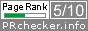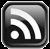# LEARN MS-ACCESS TIPS AND TRICKS

Learn Microsoft Access Advanced Programming Techniques, Tips and Tricks.

### Continued from Last Week's Post.

This is the continuation of earlier Articles:

Please go through the earlier Articles before continuing. . .

We will bring forward the result of the Decimal 255 to Binary conversion for a closer look at these two numbers: 11111111.

Decimal Number 255 needs only 3 digits to write this quantity but when we convert it into binary it needs 8 binary digits orbits.  Earlier computer programs were written using binary instructions.  Look at the example code given below:

Later programming language like Assembly Language was developed using Mnemonics (8 bit based binary instructions) like ADD, MOV, POP, etc.  Present-day Compilers for high-level languages are developed using Assembly Language. A new numbering system was devised to write binary numbers in short form.

### Octal Numbering System.

This numbering system has the number 8 as its base and is known as Octal Numbering System.  Based on the general rule that we have learned, Octal Numbering System has digits 0 to 7 (one less than the base value 8) to write numerical quantities. There are no 8 or 9 digits in this numbering system. Octal Numbering System has been devised to write Binary instructions into short form and to write program codes easily.

For example, an 8-bit Binary instruction looks like the following:

00010111  (instruction in Octal form 027), ADD B, A (Assembly Language)

The first two bits (00) represent the operation code ADD, the next three bits (010) represent CPU Register B and the next three bits (111) represent CPU Register A, the 8-bit binary instruction says to add the contents of register A to register B.  If the instruction must be changed to (ADD A, B) add register B to A then the last six bits must be altered as 00,111,010. This can be easily understood if it is written in Octal 027 to 072 rather than Binary 00111010.

So, the Octal (Base-8) Numbering System was devised to write binary-based instructions in short form. Coming back to the Octal Numbering System, let us see how we can work with these numbers.  First, we will create a table similar to the decimal/binary numbering Systems.

 85 84 83 82 81 80 32768 4096 512 64 8 1

We will use the same methods we have used for Binary to convert Decimal to Octal Numbers.

Example: Converting 255 into Octal Number?

For Binary conversion, we could take the highest integer value from the table above and subtract it from 255.  But here we cannot do that.  By looking at the table above we can see that 512 is more than 255, so we must see next number 64 goes how many times into 255?

#### Method-1:

255/64= Quotient=3, Remainder=63 (Here, we have to take the Quotient as the Octal Digit).

In this method, we must take Quotient 3 ( 64 x 3 = 192) for our result value and the balance is 63 (i.e. 255 - 192)

 85 84 83 82 81 80 32768 4096 512 64 8 1 3

63/8 = Quotient = 7, Remainder=7

 85 84 83 82 81 80 32768 4096 512 64 8 1 3 7

7 is not divisible by 8, hence 7 goes into the Units position

 85 84 83 82 81 80 32768 4096 512 64 8 1 3 7 7

#### Method-2:

255/8 = Quotient = 31, Remainder=7

 85 84 83 82 81 80 32768 4096 512 64 8 1 7

31/8 = Quotient = 3, Remainder=7

 85 84 83 82 81 80 32768 4096 512 64 8 1 7 7

3 is not divisible by 8, hence it is taken to the third digit position.

 85 84 83 82 81 80 32768 4096 512 64 8 1 3 7 7

### Writing Binary to Octal Short Form

As I mentioned earlier the Octal Numbering System has been devised to write Binary Numbers into short form.  Let us see how we can do this and convert binary numbers easily into Octal numbers.

The Decimal Number 255 when converted into Binary we get 11111111. To convert it into Octal Numbers set the binary digits into groups of three bits (011,111,111) from right to left and add up binary values of each group and write the Octal value.

011 = 1+2 = 3

111 = 1+2+4 = 7

111 = 1+2+4 = 7

Result: = 377 Octal.

You may try converting a few more numbers of your own to understand this Numbering System better.  First, convert some Decimal Numbers to Binary and group the Binary Digits in sets of 3 bits and add up the values of the group as if they are the first three bits of the binary number.

Since Octal Numbers are written with digits 0 to 7, it looks like decimal numbers to both man and machine. There must be a way to distinguish Octal Numbers from decimal numbers. Therefore it is written with the prefix characters &O. When Octal numbers are written alone or used in expressions it is written with the prefix characters &O (the letter O, not case sensitive) or &0 (digit zero), like &O0377 or &O377 or &0377.

You can try this by typing the above number in the Debug Window in the VBA Editing Screen of Microsoft Access or Excel.

Examples:

? &O0377

result: 255

? &0377

result: 255

? &0377 * 2

result: 510

Next, we will learn the Base-16 (Hexadecimal) Numbering System.

Technorati Tags:
Share:

Comments subject to moderation before publishing.

## TranslateSubscribe in a readerYour email address:

Delivered by FeedBurner